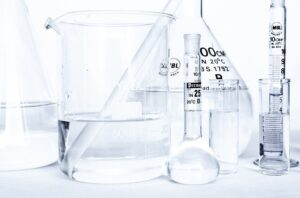# Category: A Level

Here, you will find all the resources related to A level exams. We cover A Level Math, Chemistry and Physics.

## Using Graphic Calculator for AP GP Questions ( Arithmetic and Geometric Progression)

In this post, let’s look at the use of graphic calculator for AP GP questions. You need the graphic calculator to solve some types of…## Quiz on Alkanes – H2 Chemistry

In this Quiz on alkanes written based on A Level H2 Chemistry syllabus, you’ll see the more challenging questions from this topic on alkane.## A Level H2 Chemistry Practical

Are you familiar with what’s tested in A Level H2 Chemistry Practical or H2 Chemistry Paper 4? Let’s find out in this article.## Graphic Calculator for A Level H2 Math

Having a graphic calculator for A Level H2 Math exam is a must for students. There aren’t many types of graphic calculators approved for the…## Definitions of enthalpy changes (A Level Chemistry Chemical Energetics)

Students are expected to know the definition of all the definitions of enthalpy changes tested in A Level Chemistry Chemical Energetics. While questions that involve…

## Qualitative Analysis For A Level Chemistry Practical – Testing For 2 Cations

Testing for 2 unknown cations in qualitative analysis is a common question tested in A Level Chemistry Practical, and it’s actually an easy- to- score…